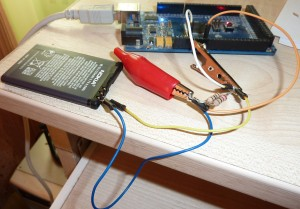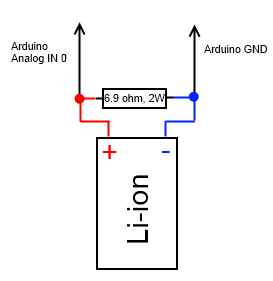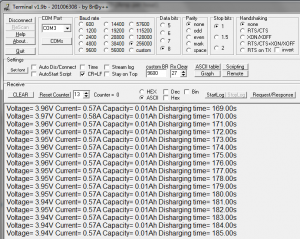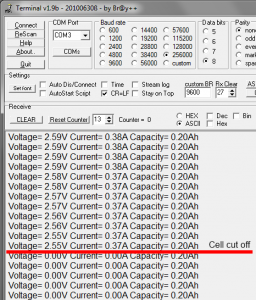# Very simple Arduino Lithium-ion battery capacity tester/discharge monitorThis is a very simple capacity tester. It consists of single resistor that discharges battery. Arduino measures the voltage drop across resistor. According to Ohm’s Law current = voltage/resistance. Every second value of current is divided by 3600 and summed up to get the capacity expressed in Ah (Amp per hour).I have used two parallel connected resistors that total resistance is 6.9 ohm. Make sure that they have proper power rating, if You don’t want them to convert to smoke. If voltage across 6.9 ohm resistor is  3.7 V, then current – 0.54 A, power ~ 2W.

Discharging of battery is manual process so please keep eye on it to make sure that battery is not overheating.

After start of discharging information about progress is transmitted via serial port and look like this.Li-ion battery voltage will drop constantly to about ~ 2V. Li-ion batteries have integrated circuit to protect a cell that cuts off circuit to prevent from over discharging.After voltage drop to 0, please disconnect battery, measurement is over.

During process of measurement please do not disconnect terminal from serial port, because Arduino could reset.

This method also can be used to measure a capacity of NiMh batteries, but these type of batteries doesn’t have IC, so user must manually stop measurement when voltage of one cell drops to 1.0 V or else batteries can be ruined.

A reference voltage of ADC of Arduino is 5V, so make sure that batteries voltage is bellow that value, or use voltage divider.

Program code bellow:

```
// Very simple Arduino Lithium-ion battery capacity tester
// from electronicsblog.net

#define LED 13
#define resistor 6.9

float capacity=0, value,voltage,current, time=0;

void measure (void) {

voltage=value/1024*5.0;

current = voltage/resistor;

capacity=capacity+current/3600;

time++;

Serial.print("Voltage= ");
Serial.print(voltage);

Serial.print("V Current= ");
Serial.print(current);

Serial.print("A Capacity= ");
Serial.print(capacity);
Serial.print("Ah ");

Serial.print("Discharging time= ");
Serial.print(time);
Serial.print("s ");

Serial.print("\n");
}

boolean x=false;

ISR(TIMER1_OVF_vect) {
TCNT1=0x0BDC;
x=!x;

measure();

}

void setup() {

pinMode(LED, OUTPUT);

TIMSK1=0x01; // enabled global and timer overflow interrupt;
TCCR1A = 0x00; // normal operation page 148 (mode0);
TCNT1=0x0BDC; // set initial value to remove time error (16bit counter register)
TCCR1B = 0x04; // start timer/ set clock

Serial.begin(256000);

};

void loop () {

digitalWrite(LED, x);

};

```

Code here is  formatted by  using Arduino Code Syntax Highlighting Plugin.